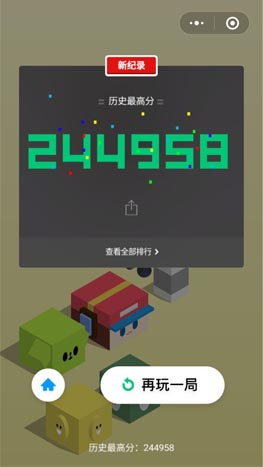@Tyhj 2018-11-13T11:18:17.000000Z 字数 5247 阅读 1798

# Android上实现微信跳一跳外挂

Android### 实现步骤### 可行性分析

#### 距离计算

##### 移动悬浮窗

//在service里面开启悬浮框代码btnView = new ImageView(getApplicationContext());        btnView.setImageResource(R.drawable.ic_star);        windowManager = (WindowManager) getApplicationContext()                .getSystemService(Context.WINDOW_SERVICE);        params = new WindowManager.LayoutParams();        // 设置Window Type        params.type = WindowManager.LayoutParams.TYPE_SYSTEM_ERROR;        // 设置悬浮框不可触摸        params.flags = WindowManager.LayoutParams.FLAG_NOT_TOUCH_MODAL                | WindowManager.LayoutParams.FLAG_NOT_FOCUSABLE | FLAG_LAYOUT_INSET_DECOR;        // 悬浮窗不可触摸，不接受任何事件,同时不影响后面的事件响应        params.format = PixelFormat.RGBA_8888;        // 设置悬浮框的宽高        params.width = 200;        params.height = 200;        params.gravity = Gravity.TOP;        params.x = 300;        params.y = 200;        windowManager.addView(btnView, params);
##### 图像识别

//模板匹配实现代码Mat template = Highgui.imread(templateFilePath1, Highgui.CV_LOAD_IMAGE_COLOR);Mat source = Highgui.imread(originalFilePath, Highgui.CV_LOAD_IMAGE_COLOR);//创建于原图相同的大小，储存匹配度Mat result = Mat.zeros(source.rows() - template.rows() + 1, source.cols() - template.cols() + 1, CvType.CV_8UC1);//调用模板匹配方法Imgproc.matchTemplate(source, template, result, Imgproc.TM_CCOEFF_NORMED);//获得最可能点，MinMaxLocResult是其数据格式，包括了最大、最小点的位置x、yCore.MinMaxLocResult mlr = Core.minMaxLoc(result);System.out.println("相似度：" + mlr.maxVal);Point matchLoc = mlr.maxLoc;//在原图上的对应模板可能位置画一个绿色矩形Core.rectangle(source, matchLoc, new Point(matchLoc.x + template.width(), matchLoc.y + template.height()), new Scalar(0, 255, 0));
##### 颜色识别

int color = bitmap.getPixel(x, y)

#### 实现自动点击

/**     * @param cmd  需要执行的adb命令     */    public static void execShellCmd(String cmd) {        try {            if (process == null) {                process = Runtime.getRuntime().exec("su");                os = new DataOutputStream(process.getOutputStream());            }            os.writeBytes(cmd + "\n");            os.flush();        } catch (IOException e) {            e.printStackTrace();        }    }

#### 获取转换公式

double k = (distence * (-0.00020) + 1.495);        if (k > 1.4165) {            k = 1.4165;        }        time = (int) (k * distence);

### 具体实现

#### 开启悬浮窗

<uses-permission android:name="android.permission.SYSTEM_ALERT_WINDOW" />//获取悬浮窗权限FloatWindowManager.getInstance().applyOrShowFloatWindow(MainActivity.this)

#### 截取屏幕

//开启录屏服务ScreenRecordUtil.getInstance().screenShot(MainActivity.this, null);//随时获取截图Bitmap bitmap = ScreenRecordUtil.getInstance().getScreenShot();

#### 颜色识别

/**     * LAB颜色空间计算色差，基于人眼对颜色的感知，     * 可以表示人眼所能感受到的所有颜色。     * L表示明度，A表示红绿色差，B表示蓝黄色差     */    public static int labAberration(int color1, int color2) {        int r1 = Color.red(color1); // 取高两位        int g1 = Color.green(color1);// 取中两位        int b1 = Color.blue(color1);// 取低两位        int r2 = Color.red(color2); // 取高两位        int g2 = Color.green(color2);// 取中两位        int b2 = Color.blue(color2);// 取低两位        int rmean = (r1 + r2) / 2;        int r = r1 - r2;        int g = g1 - g2;        int b = b1 - b2;        return (int) Math.sqrt((2 + rmean / 256) * (Math.pow(r, 2)) + 4 * (Math.pow(g, 2)) + (2 + (255 - rmean) / 256) * (Math.pow(b, 2)));    }

#### 找棋子位置#### 获取方块的中心##### 方块不是纯色##### 特殊的纯色#### 一些需要注意的地方#### 自我完善

CrashHander.getInstance().init(this, new ISaveErro() {            @Override            public void saveErroMsg(Throwable throwable) {            }        });• 私有
• 公开
• 删除